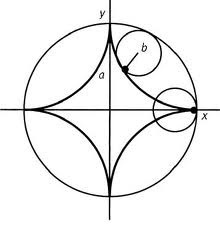## Hypocycloid Assignment Help

Assignment Help: >> Geometrical Construction - Hypocycloid

Hypocycloid:

Whenever a circle of diameter D rolls on the inside of another circle of radius R, the path of the point A is like on the rolling circle is called a hypocycloid as displayed in diagram.To sketch the hypocycloid curve the construction is rather similar to such of the epicycloids.

Describe a rolling circle of diameter D and a guiding circle of radius R tangent to it at point A. Divide the angle α such that is equal to 180 D/R and the rolling and guiding circles into 12 equal parts. From O0 and via the points of division on the guiding circle, sketch radial lines that will intersect the auxiliary circle of radius R - D/2 at point O1 via O12.

Along with O0 as a center, describe auxiliary arcs passing through the points of division D/2 of the rolling circle. From O1 via O12 like centers and along with a radius equal to sketch arcs to intersect the corresponding auxiliary arcs at the points A1 via A12 of the desired hypocycloid. A smooth curve is then displayed through these points to get the desired hypocycloid.